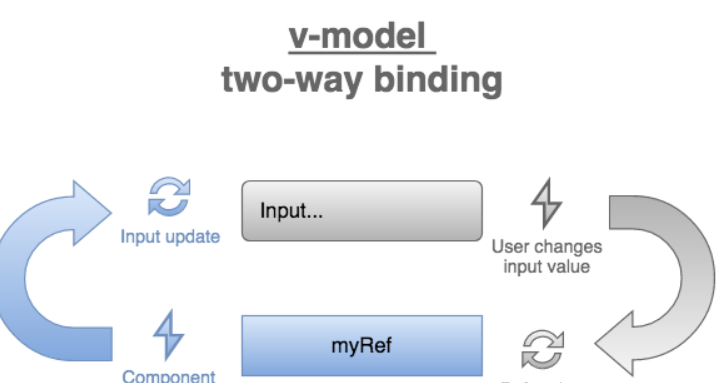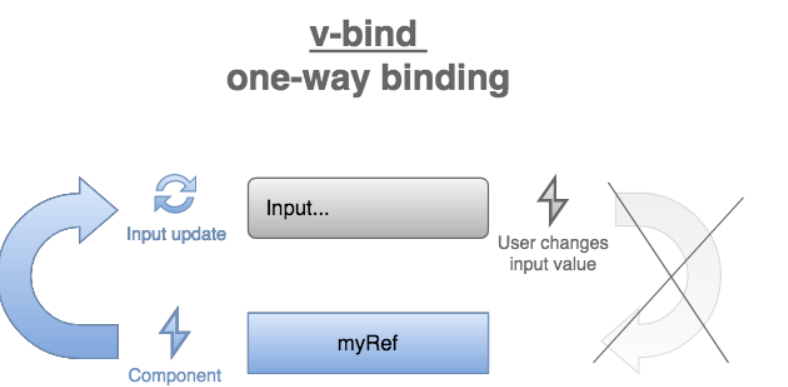• Vue双向绑定表单实例
• 发布于 2个月前
• 65 热度
0 评论
Vue中v-model的思路很简单。定义一个可响应式的text（通常是一个ref），然后用v-model="text"将这个值绑定到一个input上。这就创造了一个双向的数据流：
1.用户在输入框中输入，text会发生变化。
2.text发生变化，输入框的值也随之变化。

1. const text = ref()：作为v-model可响应式的值。
2. v-model="text"：将v-model添加到分配有text的输入表单标签中。
```<script setup>
import { ref } from 'vue'
const text = ref('Unknown') // Step 1: create data bus
</script>
<template>
<!-- Step 2: assign data bus to v-model -->
<input v-model="text" type="input" />
<div>{{ text }}</div>
</template>```

"v-model="text" 在Vue中属于双向绑定数据。"v-model与v-bind

`<input v-bind:value="text" type="text" />`

`<input :value="text" type="text" />`
v-model和:value的不同之处是什么？<input :value="value" />是一种单向数据流机制。

```<script setup>
import { ref } from 'vue'
const text = ref('Unknown')
</script>
<template>
<input :value="text" type="input" />
<div>{{ text }}</div>
</template>````<input v-model="text" type="text" />`

`<input :value="text" @input="text = \$event.target.value" type="text" />`

```<script setup>
import { ref } from 'vue'
const text = ref('Unknown')
</script>
<template>
<input
:value="text"
@input="text = \$event.target.value"
type="input"
/>
<div>{{ text }}</div>
</template>```

reactive()是Vue里的响应式API，可以让对象具有响应式。ref()和reactive()的最大不同点就是，ref()可以存储原始值和对象，而reactive()值接受对象。并且reactive()对象可以直接访问，而不需要像ref()那样需要通过.value属性访问。

```<script setup>
import { reactive } from 'vue'
const person = reactive({ firstName: 'John', lastName: 'Smith' })
</script>
<template>
<input v-model="person.firstName" type="input" />
<input v-model="person.lastName" type="input" />
<div>Full name is {{ person.firstName }} {{ person.lastName }}</div>
</template>```
const person = reactive({ firstName: '', lastName: '' })创建了一个响应式的对象。
v-model="person.firstName"与名字属性绑定，以及v-model="person.lastName"与姓氏属性绑定。

Textarea

```<script setup>
import { ref } from 'vue'
const longText = ref("Well... here's my story. One morning...")
</script>
<template>
<textarea v-model="longText" />
<div>{{ longText }}</div>
</template>```
Select
select也就是下拉框，为用户提供了一组预定义的选项供其选择。

```<script setup>
import { ref } from 'vue'
const employeeId = ref('2')
</script>
<template>
<select v-model="employeeId">
<option value="1">Jane Doe</option>
<option value="2">John Doe</option>
<option value="3">John Smith</option>
</select>
<div>Selected id: {{ employeeId }}</div>
</template>```
employeeId是与select绑定的ref，将获得被选择的选项的值。

```<script setup>
import { ref } from 'vue'
const employee = ref('Jane Doe')
</script>
<template>
<select v-model="employee">
<option>Jane Doe</option>
<option>John Doe</option>
<option>John Smith</option>
</select>
<div>Selected: {{ employee }}</div>
</template>```

Checkbox

`<input ref="checked" type="checkbox" />`
checked被赋予一个布尔值，表示该复选框是否被选中。

```<script setup>
import { ref } from 'vue'
const checked = ref(true)
</script>
<template>
<label><input v-model="checked" type="checkbox" />Want a pizza?</label>
<div>{{ checked }}</div>
</template>```
checked的初始值是true，因此复选框默认是被选中的。勾选或不勾选复选框，会相应地将checked更新为true或false。为了将勾选或不勾选绑定到布尔值以外的其他自定义值，Vue在复选框上提供了2个Vue特有的属性：
```<input
v-model="checked"
true-value="Yes!"
false-value="No"
/>```

```<script setup>
import { ref } from 'vue'
</script>
<template>
<label>
<input v-model="answer" type="checkbox" true-value="Yes!" false-value="No"  />
Want a pizza?
</label>
</template>```

```<input type="radio" v-model="option" value="a" />

```<script setup>
import { ref } from "vue"
const color = ref("white")
</script>
<template>
<div>T-shirt color: {{ color }}</div>
</template>```

```<script setup>
import { ref } from "vue"
const color = ref("white")
const COLORS = [
{ option: "white", label: "White" },
{ option: "black", label: "Black" },
{ option: "blue", label: "Blue" },
]
</script>
<template>
<label v-for="{ option, label } in COLORS" :key="option">
<input type="radio" v-model="color" :value="option" /> {{ label }}
</label>
<div>T-shirt color: {{ color }}</div>
</template>```
v-model修饰符

trim

v-model.trim修饰符在赋值给绑定的ref之前清除输入表单的值：
```<script setup>
import { ref } from 'vue'
const text = ref('')
</script>
<template>
<input v-model.trim="text" type="text" />
<pre>"{{ text }}"</pre>
</template>```
number
v-model.number修饰符在输入表单的值上应用一个数字解析器。如果用户引入了一个可以解析为数字的值，v-model.number="number"则将解析后的数字分配给number。在其他情况下，如果引入的值不是数字，number就会被分配为原始字符串。
```<script setup>
import { ref } from "vue";
const number = ref("");
</script>
<template>
<input v-model.number="number" type="text" />
<div>{{ typeof number }}</div>
</template>```

lazy

input和change事件的主要区别是什么呢？
1. input是每当你在输入表单键入时就会触发。
2. change是只有当你把焦点从输入表单移开时，才会触发。在输入表单里输入并不会触发change事件。

```<script setup>
import { ref } from 'vue'
const text = ref('Unknown')
</script>
<template>
<input v-model.lazy="text" type="input" />
<div>{{ text }}</div>
</template>```

v-model将表单输入与ref或响应式对象进行绑定。